# Weird Maths (6 / 1.5)

## Recommended Posts

I had to divide 6 through 1.5, so I tried to do it the easy way:

6 / 1 = 6

6 / 2 = 3

The logical assumption would be:

6 / 1.5 = 4.5

Boy, I was wrong!  O_o

6 / 1.5 = 4

Yes, that's right, the correct answer is 4! I mean, it's pretty logical if you think about it. Anyone else finds this confusing though?  :laugh:

##### Share on other sites

Yes, that's right, the correct answer is 4! I mean, it's pretty logical if you think about it. Anyone else finds this confusing though?  :laugh:

No thats just basic Maths.

Its not that hard

##### Share on other sites

Well, yeah. It's not hard for the calculator. But I tried doing it without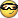and failed.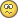##### Share on other sites

Well, yeah. It's not hard for the calculator. But I tried doing it withoutand failed.Maybe you should stick to doing things with calculators available (which is almost everywhere at least)

##### Share on other sites

X+X=X*X

There are 2 answers to be expected from this math problem and other people to your post.

I'll give you the lower number, while you expect people responding with the latter answer.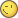##### Share on other sites

X+X=X*X

There are 2 answers to be expected from this math problem and other people to your post.

I'll give you the lower number, while you expect people responding with the latter answer.x +x = x * x

2x = x²

0 = x² -2x

x² -2x = 0

0 or 2 right?

Im assuming thats x(x-2)=0

x² -2x = 0

x(x -2) = 0

x1 = 0; x2 = 2

Correct. That's a quadratic function! But what does this have to do with the topic? The topic is about weird, confusing division rules!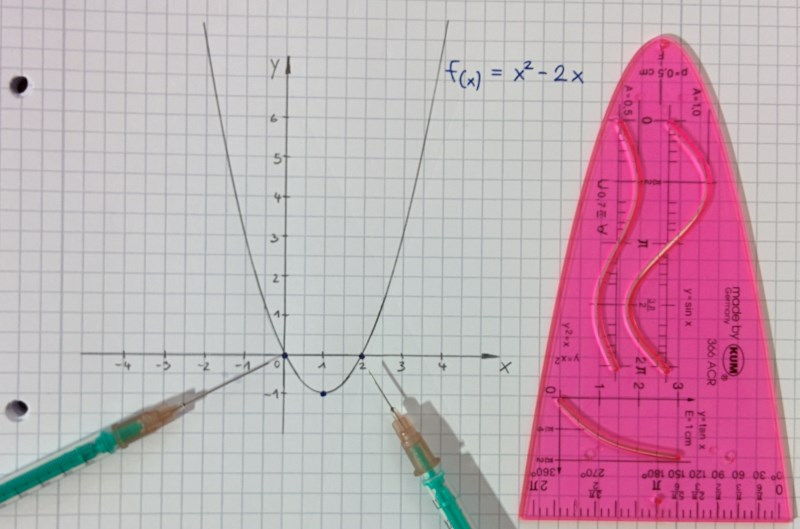##### Share on other sites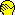Nothing to be ashamed about, bro, IMHO this is a valid topic.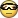##### Share on other sites

wtf, man. Seriously. wtf.

um.... yeah.... 60/15=4  and I'm no programmer.  Am I missing something?

Or just multiply both parts by 2, and get 6/1.5 = 12/3 = 4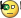##### Share on other sites

wtf, man. Seriously. wtf.

Well, my basic maths is not quite up to snuff.##### Share on other sites

ugh, alright then, I explain the previous post of mine.

Answer 0, you get a 0 for not knowing.

Answer 2. others could say, hey; me two don't know.

I should probably take humour classes in the near future.

6 is my favourite math number. You can do all kind of math tricks with it.

1+2+3=1*2*3

stuff like that.

##### Share on other sites

42 is best cause it's the answer to everything

##### Share on other sites

X+X=X*X

There are 2 answers to be expected from this math problem and other people to your post.

I'll give you the lower number, while you expect people responding with the latter answer.or in other words, 0, 2

Rearranging stupidly cause well it's fun, only works on 0 and 2 also.

2x = x^2

x = ( ( x^2 ) / 2)

Alternatively you could just realize this is a quadratic form also.

ax^2 + bx + C = 0

( x^2 - 2x ) = 0

factor out the x

x ( x - 2 ) = 0

##### Share on other sites

Thanks X3M for derailing a topic about weird division rules into a topic about quadratic functions.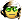##### Share on other sites

I had to divide 6 through 1.5, so I tried to do it the easy way:

6 / 1 = 6

6 / 2 = 3

The logical assumption would be:

6 / 1.5 = 4.5

Boy, I was wrong!  O_o

6 / 1.5 = 4

Yes, that's right, the correct answer is 4! I mean, it's pretty logical if you think about it. Anyone else finds this confusing though?  :laugh:

It's weird to you because you're looking at your little sequence like it's multiplication, and not division which it is. Let me extend the sequence to include your 'weird' assumption:

6 / 1 = 6

6 / 1.5 = 4.5    <-- Alex's anomaly

6 / 2 = 3

You've assumed that dividing 6 by what is HALFWAY between 1 and 2 (which is 1.5) would equal what is HALFWAY between the answers 6 and 3 (which is 4.5), which is obviously incorrect. Now, if it were a multiplication sequence/pattern, then it'll make sense:

6 x 1 = 6

6 x 1.5 = 9

6 x 2 = 12

In this case, MULTIPLYING by what is HALFWAY between 1 and 2 (which is 1.5) does equal what is HALFWAY between the answers 6 and 12 (which is 9). It works with any other sequence. For example:

10 x 10 = 100

10 x 15 = 150

10 x 20 = 200

or

10 x 1.5 = 15

10 x 2 = 20

10 x 2.5 = 25

or whatever. But I do admit that at a glance, especially because of the way you've described it, it did look kinda weird.

##### Share on other sites

X+X=X*X

There are 2 answers to be expected from this math problem and other people to your post.

I'll give you the lower number, while you expect people responding with the latter answer.x +x = x * x

2x = x²

0 = x² -2x

x² -2x = 0

0 or 2 right?

Im assuming thats x(x-2)=0

x² -2x = 0

x(x -2) = 0

x1 = 0; x2 = 2

Correct. That's a quadratic function! But what does this have to do with the topic? The topic is about weird, confusing division rules!

That is correct only if your algebra is a domain of integrity. (a*b = 0 <=> a = 0 or b = 0) Take, for instance, the ring (Mn®, +, *). Then X(X - 2I) = 0 do not imply that X = 0 or X - 2I = 0 are the only solutions. Take the matrix:

[ 2  0 ]

[ 0  0 ]

It is different from 0 and 2*I and still solves your equation.

##### Share on other sites

Thanks Brian, that's the kind of post I've been looking for!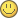Haha, thanks for naming it "Alex's anomaly" - maybe they'll teach this to first or second graders in school and have some good laughs of how stupid I am in a couple of years time. I'd really love having a math problem named after me!So, if:

```6 / 1    = 6
6 / 1.5  = 4
6 / 2    = 3
```

I really wonder what...

```6 / X = 4.5
```

would be?

Let's find out!

```6 / X      = 4.5           | * X
6          = 4.5X          | * 2
12         = 9X            | / 9
12 / 9     = X
4 / 3      = X
1.3333333  = X
```

Note that there's actually a period on 1.3 (as if this wasn't confusing enough).  :laugh:  So:

```6 / 1           = 6
6 / 1.2         = 5
6 / 1.3333333   = 4.5
6 / 1.5         = 4
6 / 2           = 3
```

I extended this with 1.2, too, which is interesting - but completely illogical! Weird as phuck! Damn you, maths!!!  :ranting:

##### Share on other sites

I actually drew the graph of f(x) = 6 / x!  :heady:

Any math teacher would probably slap me for connecting the dots in a straight line.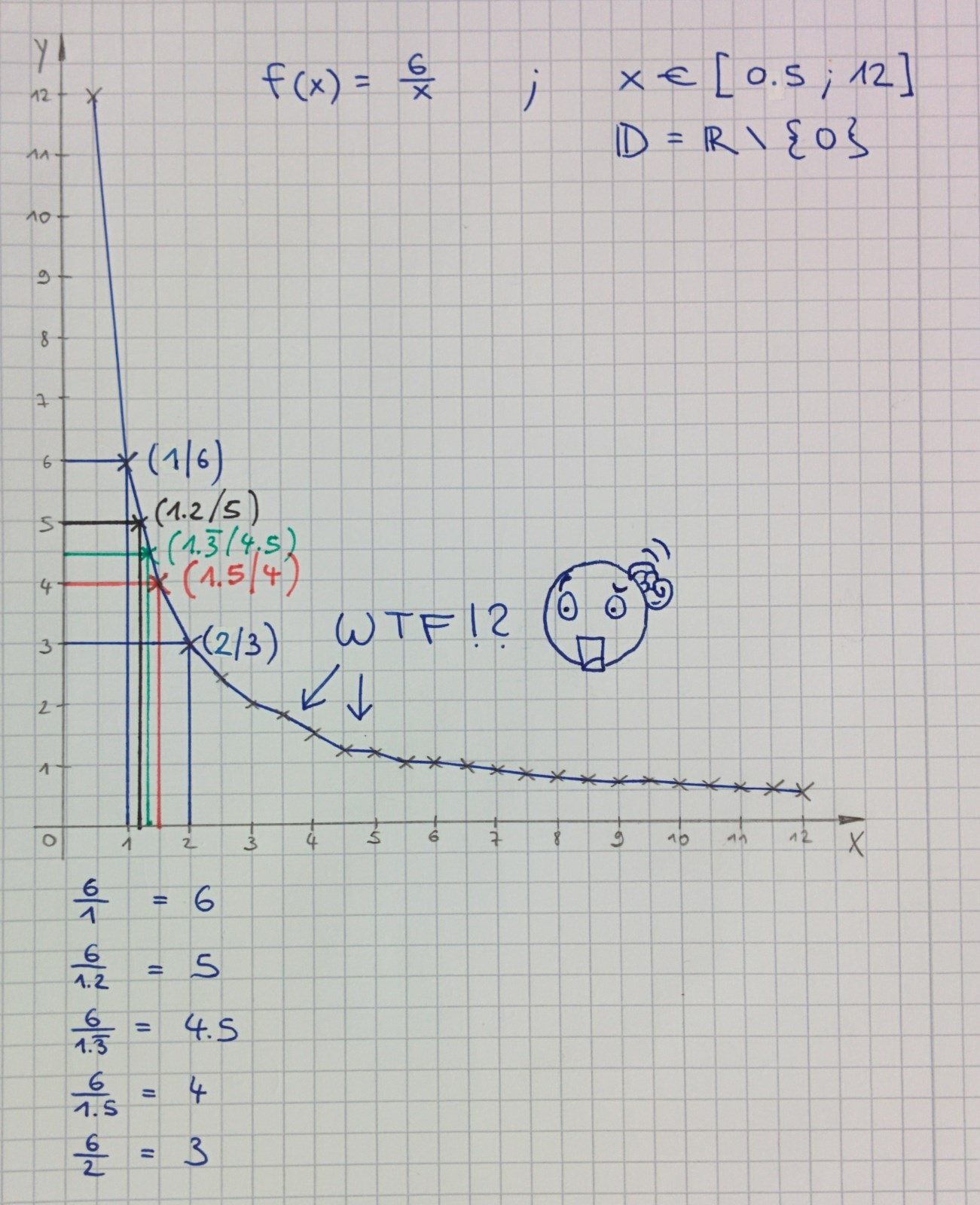##### Share on other sites

Thanks, I figured it out. Division sucks and curves are hella confusing! Did you see what happens when you're dealing with negative numbers?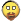x > 0 and dividend > 0 ---> 1st quadrant

x > 0 and dividend < 0 ---> 2nd quadrant  :ranting:

x < 0 and dividend > 0  ---> 3rd quadrant  :ranting:

x < 0 and dividend < 0 ---> 4th quadrant  :ranting:

It basically mirrors the graph into any of the said quadrants (pretty sure this is correct, somebody check please).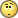##### Share on other sites

SiRaLex: You should check this tool:

you can plot graphs, derivate functions, integrate, solve linear systems, etc.

Pichorra, thank you so much! This shall help me in my quest to master the art of mathematics!But for now...

Hilary Duff - The Math

xD

## Create an account

Register a new account